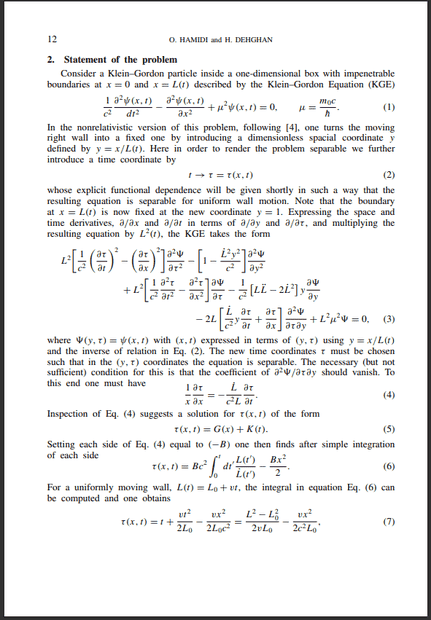# Separation of Variables, but not equal to constant

• I
• Foracle

#### Foracle

TL;DR Summary
Is the following PDE separable
$$\frac{1}{T} \frac{\partial T}{\partial t} = k \frac{1}{Q} \frac{\partial Q}{\partial q} - \frac{\partial q}{\partial t} \frac{1}{Q} \frac{\partial Q}{\partial q}$$
where ##\frac{\partial q}{\partial t}## can depend on ##q## and ##t##?
Suppose I have 2 variables q and t (time), where q is some reparameterization of x (position) : ##x \to q = x f(t)##.

Suppose I have a partial differential equation :
$$\frac{\partial u(q,t)}{\partial t} = k \frac{\partial u(q,t)}{\partial q}$$
where k = constant
Then I do a separation of variables ## u(q,t) = Q(q)T(t) ##
The differential equation becomes (after some manipulation):
$$\frac{1}{T} \frac{\partial T}{\partial t} = k \frac{1}{Q} \frac{\partial Q}{\partial q} - \frac{\partial q}{\partial t} \frac{1}{Q} \frac{\partial Q}{\partial q}$$
where I have used the fact that ##\frac{\partial Q}{\partial t} = \frac{\partial q}{\partial t} \frac{\partial Q}{\partial q}##

Now the left hand side is only dependent on ##t##, while the right hand side depends on both ##q## and ##t##. Since both sides still depend on ##t##, can I say that
$$(LHS) = (RHS) = g(t)$$
(LHS = Left hand side, RHS = Right hand side, g(t) is some function of time)

I have seen on a research paper where the author says that for the above equation to be separable, ##\frac{\partial q}{\partial t}## has to be constant so that RHS only depends on ##q##, hence ##(LHS) = (RHS) = constant##.
But since ##q## still depends on time (##q = x f(t)##), doesn't this mean RHS still depends on time and it should be ##(LHS) = (RHS) = g(t)## instead?

Last edited:

Summary:: Is the following PDE separable
$$\frac{1}{T} \frac{\partial T}{\partial t} = k \frac{1}{Q} \frac{\partial Q}{\partial q} - \frac{\partial q}{\partial t} \frac{1}{Q} \frac{\partial Q}{\partial q}$$
where ##\frac{\partial q}{\partial t}## can depend on ##q## and ##t##?

Suppose I have 2 variables q and t (time), where q is some reparameterization of x (position) : ##x \to q = x f(t)##.

Suppose I have a partial differential equation :
$$\frac{\partial u(q,t)}{\partial t} = k \frac{\partial u(q,t)}{\partial q}$$
where k = constant
Then I do a separation of variables ## u(q,t) = Q(q)T(t) ##
The differential equation becomes (after some manipulation):
$$\frac{1}{T} \frac{\partial T}{\partial t} = k \frac{1}{Q} \frac{\partial Q}{\partial q} - \frac{\partial q}{\partial t} \frac{1}{Q} \frac{\partial Q}{\partial q}$$
where I have used the fact that ##\frac{\partial Q}{\partial t} = \frac{\partial q}{\partial t} \frac{\partial Q}{\partial q}##

If by $\dfrac{\partial}{\partial t}$ you mean differentiation with respect to $t$ with $q$ held constant, then by definition $$\frac{\partial q}{\partial t} = 0$$ and $Q$ depends on $q$ alone.

Now the left hand side is only dependent on ##t##, while the right hand side depends on both ##q## and ##t##. Since both sides still depend on ##t##, can I say that
$$(LHS) = (RHS) = g(t)$$
(LHS = Left hand side, RHS = Right hand side, g(t) is some function of time)

I have seen on a research paper where the author says that for the above equation to be separable, ##\frac{\partial q}{\partial t}## has to be constant so that RHS only depends on ##q##, hence ##(LHS) = (RHS) = constant##.
But since ##q## still depends on time (##q = x f(t)##), doesn't this mean RHS still depends on time and it should be ##(LHS) = (RHS) = g(t)## instead?

You need to provide more context. Are you changing variables from $(x,t)$ to $(q = xf(t),t)$? If so, differentiation with respect to $t$ with $x$ held constant is not the same thing as differentiation with respect to $t$ with $q$ held constant, and you need to be clear which of the two you mean when you write $\dfrac{\partial}{\partial t}$.

Are you changing variables from (x,t) to (q=xf(t),t)?
Yes, I am doing that.
you need to be clear which of the two you mean when you write ##\frac{∂}{∂t}##
When I do ##\frac{\partial u(q,t)}{\partial t}##, I am not holding anything constant. So
$$\frac{\partial }{\partial t} = \frac{\partial q}{\partial t} \frac{\partial}{\partial q} + \frac{\partial}{\partial t}$$
The ##\frac{\partial}{\partial t}## on the 2nd term of the RHS holds q constant.

I have another question that is kind of related.I am currently studying this research paper by O. Hamidi. What bothers me is in the equation (5), why did he choose to write ##\tau (x,t) = G(x) + K(t)## instead of the usual separation of variables ##\tau (x,t) = G(x)K(t)##?
Both substitutions give different solution, and both methods seem good to me. Why do people use one over the other?